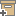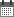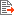Syntax
Search...
Manage
Community:
אסיף מאגר המחקר החקלאי
A theoretical study of the estimation of the correlation scale in spatially variable fields: 1. Stationary fieldsBack to searchPrevious item
Next item
Year:
1987
Source of publication :
Water Resources Research
Authors :
Russo, David
;
.
Volume :
23
Co-Authors:
Russo, D.
Jury, W.A.
Facilitators :
From page:
1257
To page:
1268
(
Total pages:
12
)
Abstract:
The correlation scale of the stochastic component of various soil properties plays an essential role in the interpolation of spatial phenomena using kriging techniques and in the solution of transport problems in heterogeneous media using stochastic modeling. In this study the uncertainty of the estimation of the correlation scales of stationary fields was analyzed by generating realizations of a set of two‐dimensional isotropic second‐order stationary functions with given correlation scales. Results from simulations of 100 independent realizations showed that the variance of the estimate of the correlation scale decreases as the number of the sample points n increases and when the size of the correlation scale of the underlying process relative to the field area decreases, whereas the biasedness decreases as n increases arid the underlying correlation scale increases. Results of variogram model fitting and cross‐validation tests involving single realizations showed that both the accuracy of the estimates of the correlation scales and the fitted variogram model consistency increase as both the sample points and the correlation scale of the underlying process increase. Analysis of the effect of the grid size on the estimates of the correlation scale showed that reasonable estimates can be obtained when the distance between the sampling points is smaller than half the range of the underlying process. Estimates of correlation scales based on data along transects may underestimate the correlation scale of the underlying process by a factor of 2 or greater. A comparison between different estimation procedures showed that in general estimates of both the variance Ĉ (0) and the integral scale Ĵ provided by the restricted maximum likelihood procedure are higher than those provided by the maximum likelihood procedure, which, in turn, are higher than those provided by fitting the nonparametric estimates of the variogram using the minimum mean‐square deviation criterion. Results of the objective model validation tests, however, implied that generally all these estimates are accurate and consistent with the data, suggesting that a given realization of a given stationary process may be adequately described by different sets of Ĵ and Ĉ(0). This may not affect the results of kriging estimation which requires the ratio C(0)/J, but may pose problems in stochastic transport modeling which requires the values of Ĵ and Ĉ(0) individually. Copyright 1987 by the American Geophysical Union.
Note:
Related Files :
FIELD SOILS
INHERENT SPATIAL VARIABILITY
Sampling
Soils
STOCHASTIC TRANSPORT MODELING
Related Content
More details
DOI :
10.1029/WR023i007p01257
Article number:
Affiliations:
Database:
Scopus
Publication Type:
article
;
.
Language:
English
Editors' remarks:
ID:
28039
Last updated date:
02/03/2022 17:27
Creation date:
17/04/2018 00:36Scientific Publication
A theoretical study of the estimation of the correlation scale in spatially variable fields: 1. Stationary fields
23
Russo, D.
Jury, W.A.
A theoretical study of the estimation of the correlation scale in spatially variable fields: 1. Stationary fields
The correlation scale of the stochastic component of various soil properties plays an essential role in the interpolation of spatial phenomena using kriging techniques and in the solution of transport problems in heterogeneous media using stochastic modeling. In this study the uncertainty of the estimation of the correlation scales of stationary fields was analyzed by generating realizations of a set of two‐dimensional isotropic second‐order stationary functions with given correlation scales. Results from simulations of 100 independent realizations showed that the variance of the estimate of the correlation scale decreases as the number of the sample points n increases and when the size of the correlation scale of the underlying process relative to the field area decreases, whereas the biasedness decreases as n increases arid the underlying correlation scale increases. Results of variogram model fitting and cross‐validation tests involving single realizations showed that both the accuracy of the estimates of the correlation scales and the fitted variogram model consistency increase as both the sample points and the correlation scale of the underlying process increase. Analysis of the effect of the grid size on the estimates of the correlation scale showed that reasonable estimates can be obtained when the distance between the sampling points is smaller than half the range of the underlying process. Estimates of correlation scales based on data along transects may underestimate the correlation scale of the underlying process by a factor of 2 or greater. A comparison between different estimation procedures showed that in general estimates of both the variance Ĉ (0) and the integral scale Ĵ provided by the restricted maximum likelihood procedure are higher than those provided by the maximum likelihood procedure, which, in turn, are higher than those provided by fitting the nonparametric estimates of the variogram using the minimum mean‐square deviation criterion. Results of the objective model validation tests, however, implied that generally all these estimates are accurate and consistent with the data, suggesting that a given realization of a given stationary process may be adequately described by different sets of Ĵ and Ĉ(0). This may not affect the results of kriging estimation which requires the ratio C(0)/J, but may pose problems in stochastic transport modeling which requires the values of Ĵ and Ĉ(0) individually. Copyright 1987 by the American Geophysical Union.
Scientific Publication
You may also be interested in